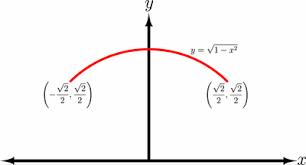# Math.atan() help

This is a pretty simple post. I cannot find the answer on the devhub so I came here.

All I want is for someone to explain what math.atan() is and does.

All of these are trigonometric functions, to get into trigonometry, you need to know algebra, I recommend learning in khan academy because it has a search bar and it quizzes you at the end.

1 Like

How fun, complicated math. Ah well, I will try it. Thanks for helping me out.

Oh, can I ask what arc means? Some other math functions return the arc of something.wiki

1 Like

This is a arc θ, the simplest way I can explain it, it is a curveIf you look at imAlex’s post the curves are the red

1 Like